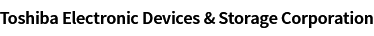### Cross Reference Search

About information presented in this cross reference

The information presented in this cross reference is based on TOSHIBA's selection criteria and should be treated as a suggestion only. Please carefully review the latest versions of all relevant information on the TOSHIBA products, including without limitation data sheets and validate all operating parameters of the TOSHIBA products to ensure that the suggested TOSHIBA products are truly compatible with your design and application.
Please note that this cross reference is based on TOSHIBA's estimate of compatibility with other manufacturers' products, based on other manufacturers' published data, at the time the data was collected.
TOSHIBA is not responsible for any incorrect or incomplete information. Information is subject to change at any time without notice.

### Stock Check & Purchase

Select Product Categories

Select Application

Find everything you need for your next product design. Simply select an application and click through to the block diagram to discover our semiconductor solutions.

New Products / News

Design & Development

Knowledge

# How to calculate the allowable power dissipation of a bias resistor built-in transistor (BRT)

The power dissipation of a BRT is the sum of the power dissipated by the internal transistor (Q) and the built-in bias resistors (R1 and R2).

Here, let’s calculate the power dissipated by the BRT shown in Figure 1 when it is in the “on” state.
Suppose that the BRT is the RN1402 (R1=R2=10 kΩ), the input voltage (VI) is 10 V, the collector-emitter voltage (VCE) is 0.2 V, the base-emitter voltage (Vbe) is 0.7 V, and the operating current gain (hFE) of the internal transistor is 10.

Then, IB, IR2, Ib, and IC are calculated as follows:
IB = ( VI – Vbe ) / R1 = ( 10 – 0.7 ) / 10 = 0.93 mA
IR2 = Vbe / R2 = 0.7 / 10 = 0.07 mA
Ib = IB – IR2 = 0.93 – 0.07 = 0.86 mA
IC = Ib * hFE = 0.86 * 10 = 8.60 mA

Let the power dissipation of R1 and R2 be PR1 and PR2 respectively and the collector-emitter and base-emitter power dissipation of the transistor be PCE and Pbe respectively.
R1: PR1 = ( VI – Vbe ) * IR1 = ( 10 – 0.7) * 0.93 = 8.65 mW
R2: PR2 = Vbe * IR2 = 0.7 * 0.007 = 0.05 mW
VCE: PCE = VCE * IC = 0.2 * 8.6 = 1.72 mW
Vbe: Pbe = Vbe * Ib = 0.7 * 0.86 = 0.60 mW

Hence, the power dissipation (P) of the BRT is calculated as:
P = PR1 + PR2 + PCE + Pbe = 11.02 mW

A new window will open
Site Map

Semiconductor Products

Storage Products

Other Products

Company